# 前言

sklearn预测评估指标：混淆矩阵计算详解-附Python计算代码

# 一、准确率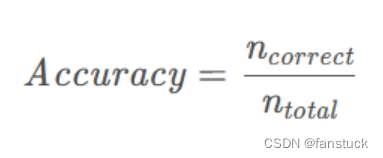```from sklearn.metrics import accuracy_score print('Accuracy:{}'.format(accuracy_score(y_true, y_pred)))```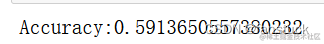# 二、精确率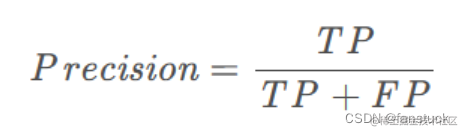sklearn的参数有：

```sklearn.metrics.precision_score(y_true, y_pred, *, labels=None, pos_label=1, average='binary', sample_weight=None, zero_division='warn')[source]```

y_true：array-like, or label indicator array / sparse matrix。真实值。

y_pred：array-like, or label indicator array / sparse matrix。预测值。 labels：array-like, default=None。当average != binary时被包含的标签集合，如果average是None的话还包含它们的顺序. 在数据中存在的标签可以被排除，比如计算一个忽略多数负类的多类平均值时，数据中没有出现的标签会导致宏平均值（marco average）含有0个组件. 对于多标签的目标，标签是列索引. 默认情况下，y_true和y_pred中的所有标签按照排序后的顺序使用. pos_labelstr or int, default=1。如果average='binary'且数据为二进制，则要报告的类。如果数据是多类或多标签的，则将忽略该数据；设置labels=[pos_label]和average！='binary”将只报告该标签的分数。

average: {‘micro’, ‘macro’, ‘samples’, ‘weighted’, ‘binary’} or None, default=’binary’。多类/多标签目标需要此参数。如果无，则返回每个类别的分数。否则，这将决定对数据执行的平均类型： - `'binary'`:仅pos_label指定的类的结果。这仅适用于目标（y_{true，pred}）为二进制的情况。

• 'micro'：通过计算总的真正例、假反例和假正例来计算全局度量。
• `'macro'`:计算每个标签的指标，并找到它们的未加权平均值。这没有考虑到标签不平衡。
• `'weighted'`:为每个标签计算指标，并通过各类占比找到它们的加权均值（每个标签的正例数）.它解决了’macro’的标签不平衡问题；它可以产生不在精确率和召回率之间的F-score.
• `'samples'`:计算每个实例的度量，并找到它们的平均值（仅对多标记分类有意义，因为这与accuracy_score不同）。

sample_weight： array-like of shape (n_samples,), default=None。标签权重

zero_division： 设置除零时返回的值。如果设置为“warn”，则该值将作为0，但也会引发警告。

```from sklearn.metrics import precision_score print('Percosopn:{}'.format(precision_score(y_true, y_pred, average='weighted')))```

Macro Average

• 如果每个类别的样本数量差不多，那么宏平均和微平均没有太大差异

• 如果每个类别的样本数量差异很大，那么注重样本量多的类时使用微平均，注重样本量少的类时使用宏平均

• 如果微平均大大低于宏平均，那么检查样本量多的类来确定指标表现差的原因
• 如果宏平均大大低于微平均，那么检查样本量少的类来确定指标表现差的原因

# 三、召回率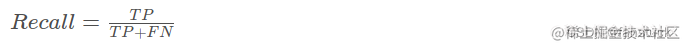```

from sklearn.metrics import recall_score print('Recall_score:{}'.format(recall_score(y_true, y_pred, average='weighted'))) ```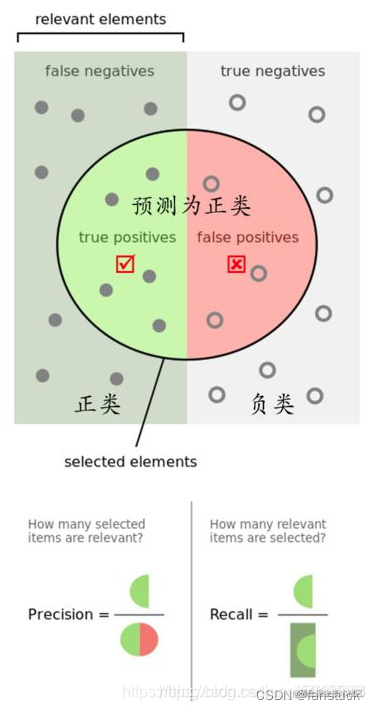# 四、[F1-score]

F1 score是精确率和召回率的调和平均值，计算公式为：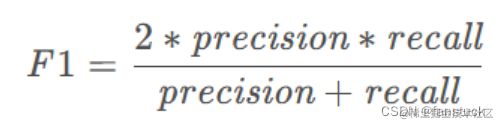Precision体现了模型对负样本的区分能力，Precision越高，模型对负样本的区分能力越强；Recall体现了模型对正样本的识别能力，Recall越高，模型对正样本的识别能力越强。F1 score是两者的综合，F1 score越高，说明模型越稳健。

```from sklearn.metrics import f1_score print('F1_socre:{}'.format(f1_score(y_true, y_pred, average='weighted')))```